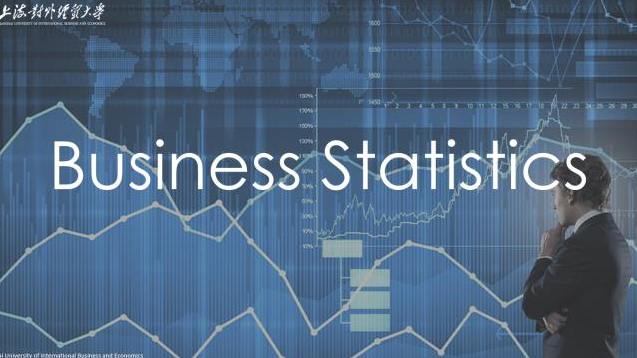Data is everywhere in business world. How to deal with the data and extract useful information from the data would be very important for decision-making. The purpose of this course is to give you, especially those in the fields of finance, management and economics and those who want to be expert in data analysis, an introduction to the fields of statistics and its applications in business. Business Statistics will help you understand the business world much more deeply.Data is everywhere in business world. How to deal with the data and extract useful information from the data would be very important for decision-making. The purpose of this course is to give you, especially those in the fields of finance, management and economics and those who want to be expert in data analysis, an introduction to the fields of statistics and its applications in business. Business Statistics will help you understand the business world much more deeply.

#### ch01-Introduction and Data Collection

--ch01.1-Introduction

--ch01.2-Types of data

#### ch02-Presenting Data in Tables and Charts

--ch02.1-Organizing Numerical Data

--ch02.2-Organizing Categorical Data

#### ch03-Numerical Descriptive Measures

--ch03.1-Measures of central tendency

--ch03.2-Measures of variation

--ch03.3-Z score and emperical rule

--ch03.4-Shape

#### ch04-Basic Probability

--ch04.1-Basic Probability Concepts

--ch04.2-Conditional Probability

--ch04.3-Bayes' Theorem

#### ch05-Discrete Probability Distributions

--ch05.1-The probability Distribution for a discrete random variable

--ch05.2-Binomial Distribution

--ch05.3-Hypergeometric Distribution and Poisson Distribution

#### ch06-The Normal Distribution

--ch06.1-Standard Normal Distribution

--ch06.2-Finding probability in a normal distribution

--ch06.3-Assessing Normality

#### ch07-Sampling and Sampling Distributions

--ch07.1-Types of sampling methods

--ch07.2-Sampling distribution of the mean

--ch07.3-Sampling distribution of the proportion

#### ch08-Confidence Interval Estimation

--ch08.1-Confidence interval estimation for the mean-sigma known

--ch08.2-Confidence interval estimation for the mean-sigma unknown

--ch08.3-Confidence interval estimation for the proportion

--ch08.4-Determing sample size

#### ch09-Fundamentals of Hypothesis Testing: One Sample Tests

--ch09.1-Fundamentals of Hypothesis Testing Methodology

--ch09.2-Test of Hypothesis about a population mean

--ch09.3-Test of Hypothesis about a population proportion

#### ch10-Two Sample Tests and One Way ANOVA

--ch10.1-Comparing the Means of two independent populations

--ch10.2-Comparing the Means of two related populations

--ch10.3-Comparing the proportions of two independent populations

--ch10.4-F test for the difference between two variances

--ch10.5-One-Way analysis of variance

#### ch11-Simple Linear Regression

--ch11.1-Determining the simple linear regression equation

--ch11.2-Measures of variation

--ch11.3-Residual analysis and inferences about regression coefficients

--ch11.4-Pitfalls in regression

### 雷平-讲师-上海对外经贸大学-国际经贸学院### 刘永辉-教授-上海对外经贸大学-统计与信息学院### 赵倩-副教授-上海对外经贸大学-统计与信息学院### 郝程程-副教授-上海对外经贸大学-统计与信息学院